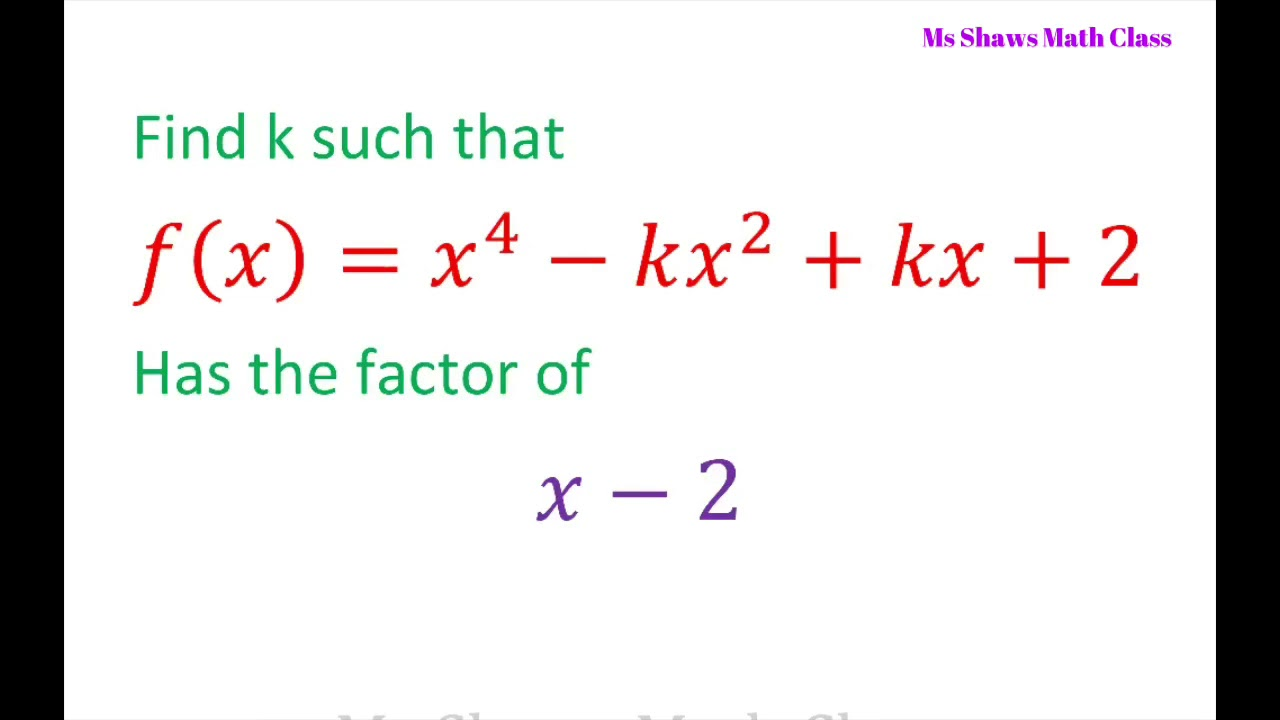d Factorize 10 x 2 − 41 x y + 21y 2 factorization applicable

# Question 2: Factorize 10 x 2 − 41 x y + 21y 2

Question 2: Factorize $$10x^2-41xy+21y^2$$

Factorization exercises , factorization examples

Solution :

To see as how to evaluate an expression for factorization, we write it;

$10x^2-41xy+21y^2$If we multiply last term i-e  ​$$21y^2$$​  with first term i-e ​$$10x^2$$​​ we get ​​$$$210 x^2y^2$ ​$$

So we break mid term i-e ​$$-41xy$$​ into two parts -35xy and -6xy  such that;

1- If we add  these two parts we get  -41xy i-e (-35xy) + ( -6xy) = -41xy

2- If we multiply these two parts we get ​$$210x^2y^2$$​​ i-e (-35xy) (-6xy) = ​$$210x^2y^2$$

So, we can write;

$=10x^2 – 35xy-6xy+21y^2$

Taking common 5x from first two terms and -3y from last two terms; we get;

$=5x(2x-7y)-3y(2x-7y)$

or

$=[5x(2x-7y)-3y(2x-7y)]$

Now (2x-7y) can be taken as common;

$=(2x-7y)[5x-3y]$

or

$=(2x-7y)(5x-3y)$